International
Tables for
Crystallography
Volume C
Mathematical, physical and chemical tables
Edited by E. Prince

International Tables for Crystallography (2006). Vol. C, ch. 8.7, pp. 722-723

## Section 8.7.3.6. Occupancies of transition-metal valence orbitals from multipole coefficients

P. Coppens,a Z. Sub and P. J. Beckerc

a732 NSM Building, Department of Chemistry, State University of New York at Buffalo, Buffalo, NY 14260-3000, USA,bDigital Equipment Co., 129 Parker Street, PKO1/C22, Maynard, MA 01754-2122, USA, and cEcole Centrale Paris, Centre de Recherche, Grand Voie des Vignes, F-92295 Châtenay Malabry CEDEX, France

#### 8.7.3.6. Occupancies of transition-metal valence orbitals from multipole coefficients

| top | pdf |

In general, the atom-centred density model functions describe both the valence and the two-centre overlap density. In the case of transition metals, the latter is often small, so that to a good approximation the atomic density can be expressed in terms of an atomic orbital basis set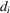, as well as in terms of the multipolar expansion. Thus,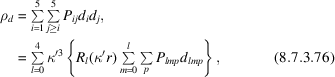in whichare the density functions.

The orbital products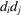can be expressed as linear combinations of spherical harmonic functions, with coefficients listed in Volume B, Chapter 1.2(ITB, 2001), which leads to relations between the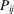and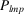. In matrix notation,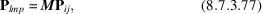where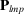is a vector containing the coefficients of the 15 spherical harmonic functions with l = 0, 2, or 4 that are generated by the products of d orbitals. The matrix M is also a function of the ratio of orbital and density-function normalization coefficients, given in Volume B, Chapter 1.2(ITB, 2001).

The d-orbital occupancies can be derived from the experimental multipole populations by the inverse expression,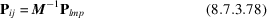(Holladay, Leung & Coppens, 1983).

The matrix M−1 is given in Table 8.7.3.3. Point-group-specific expressions can be derived by omission of symmetry-forbidden terms. Matrices for point group 4/mmm (square planar) and for trigonal point groups (3,, 32, 3m,) are listed in Tables 8.7.3.4and 8.7.3.5, respectively. Point groups with and without vertical mirror planes are distinguished by the occurrence of both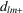and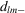functions in the latter case, and onlyin the former, with m being restricted to n, the order of the rotation axis. The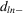functions can be eliminated by rotation of the coordinate system around a vertical axis through an anglegiven by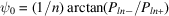.

 Table 8.7.3.3| top | pdf | The matrix M−1 relating d-orbital occupancies Pij to multipole populations Plm (from Holladay, Leung & Coppens, 1983)
d-orbital populationsMultipole populations
P00P20P22+P40P42+P44+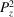0.200 1.039 0.00 1.396 0.00 0.00
Pxz 0.200 0.520 0.942 −0.931 1.108 0.00
Pyz 0.200 0.520 −0.942 −0.931 −1.108 0.00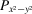0.200 −0.039 0.00 0.233 0.00 1.571
Pxy 0.200 −1.039 0.00 0.233 0.00 −1.571
 Mixing terms.
P21P21−P22+P22−P41+P41−P42+P42−P43+P43−P44−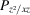1.088 0.00 0.00 0.00 2.751 0.00 0.00 0.00 0.00 0.00 0.00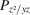0.00 1.088 0.00 0.00 0.00 2.751 0.00 0.00 0.00 0.00 0.00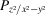0.00 0.00 −2.177 0.00 0.00 0.00 1.919 0.00 0.00 0.00 0.00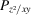0.00 0.00 0.00 −2.177 0.00 0.00 0.00 1.919 0.00 0.00 0.00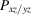0.00 0.00 0.00 1.885 0.00 0.00 0.00 2.216 0.00 0.00 0.00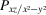1.885 0.00 0.00 0.00 −0.794 0.00 0.00 0.00 2.094 0.00 0.00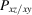0.00 1.885 0.00 0.00 0.00 −0.794 0.00 0.00 0.00 2.094 0.00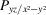0.00 −1.885 0.00 0.00 0.00 0.794 0.00 0.00 0.00 2.094 0.00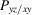1.885 0.00 0.00 0.00 −0.794 0.00 0.00 0.00 −2.094 0.00 0.00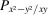0.00 0.00 0.00 0.00 0.00 0.00 0.00 0.00 0.00 0.00 3.142
 Table 8.7.3.4| top | pdf | Orbital–multipole relations for square-planar complexes (point group D4h)
P00P20P40P44+
P20 a1g 0.200 1.039 1.396 0.00
P21+ eg 0.200 0.520 −0.931 0.00
P21−   0.200 0.520 −0.931 0.00
P22+ b1g 0.200 −1.039 0.233 1.570
P22 b2g 0.200 −1.039 0.233 −1.570
 Table 8.7.3.5| top | pdf | Orbital–multipole relations for trigonal complexes
P00P2−P40P43+P43−
(a) In terms of d orbitals
P20 0.200 1.039 1.396 0.00 0.00
P21+ 0.200 0.520 −0.931 0.00 0.00
P21− 0.200 0.520 −0.931 0.00 0.00
P22+ 0.200 −1.039 0.233 0.00 0.00
P22− 0.200 −1.039 0.233 0.00 0.00
P21+/22+ 0.00 0.00 0.00 2.094 0.00
P21+/22− 0.00 0.00 0.00 0.00 2.094
P21−/22+ 0.00 0.00 0.00 0.00 2.094
P21−/22− 0.00 0.00 0.00 −2.094 0.00
(b) In terms of symmetry-adapted orbitals
P1(a1g) 0.200 1.039 1.396 0.00
P2(eg) 0.400 −1.039 −0.310 −1.975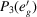0.400 0.00 −1.087 1.975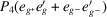0.00 −2.942 2.193 1.397
The electron density in terms of the symmetry-adapted orbitals is given by: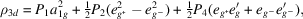with: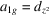;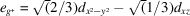;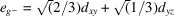;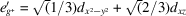; and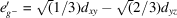.
The signs given here imply a positive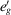lobe in the positive xz quadrant. Care should be exercised in defining the coordinate system if this lobe is to point towards a ligand atom.

### References

Holladay, A., Leung, P. C. & Coppens, P. (1983). Generalized relation between d-orbital occupancies of transition-metal atoms and electron-density multipole population parameters from X-ray diffraction data. Acta Cryst. A39, 377–387.Google Scholar
International Tables for Crystallography (2001). Vol. B, edited by U. Shmueli. Dordrecht: Kluwer Academic Publishers.Google Scholar